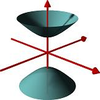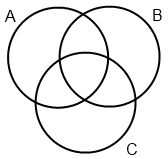#### You may also like### A Close Match

Can you massage the parameters of these curves to make them match as closely as possible?### Prime Counter

A short challenge concerning prime numbers.### The Right Volume

Can you rotate a curve to make a volume of 1?

# Venn Diagram Fun

##### Age 16 to 18 ShortChallenge Level

A Venn diagram is a way of representing all possible logical relationships between a collection of sets.

The image shows a Venn diagram for three sets $A$, $B$ and $C$How would you describe each of the seven regions in the diagram using unions $\cup$ and intersections $\cap$ of $A, B, C, A^c, B^c, C^c$ where the complements $A^c, B^c$ and $C^c$ of the sets $A, B$ and $C$ are the sets of elements not contained in $A, B$ and $C$ respectively relative to a universal set $A\cup B\cup C$

Create a Venn diagram for 4 sets $A$, $B$, $C$ and $D$. Make sure that your diagram contains regions for all possible intersections and you might like to experiment to create a particularly pleasing diagram.

Did you know ... ?

Venn diagrams are useful in many problems in logic, probability, computer science and set theory. As well as a useful tool, they are an area of study in themselves and much research has gone into the creation of particularly beautiful or symmetric Venn diagrams for larger numbers of sets.